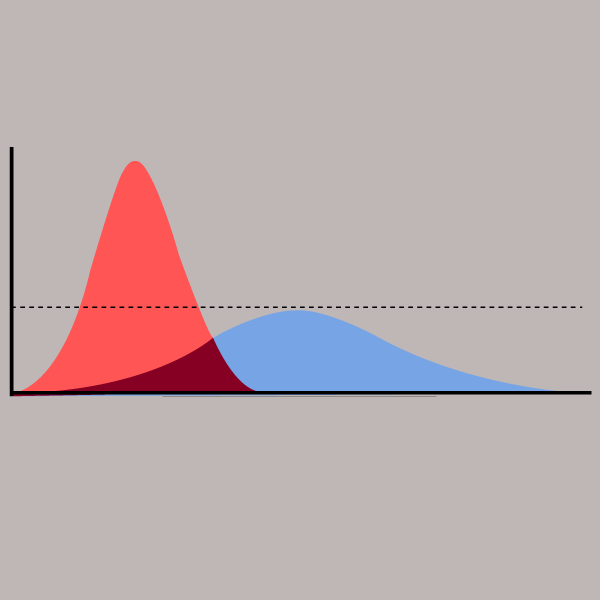# Filling an Array with a Given Value in Javascript

javascript
Published on December 21, 2019#### Use-Cases of this tutorial

• Know how to replace multiple array elements with a static value.
• Know how to create a fixed length array and fill it with a given value.

The Array.fill() method can be used to fill an array with a specific value, starting from a given index and ending upto another index.

Sometimes we may need to replace multiple elements of an array with one single value. To do this we can use the fill() method.

fill() replaces array elements from a start index to an end index with a given static value. It accepts 3 parameters :

• The value with which to replace elements.
• The start index from where the array will be filled. This is optional and by default it is set to 0 (starts from the beginning of the array).
• The end index upto and excluding which the array will be filled. This is also optional and defaults to the length of the calling array (ends upto the last element of the array).

This method changes the original array. The modified array is returned.

Using fill() is explained through some examples below.

``````var arr = [1, 2, 3, 4];

arr.fill(5);

// [5, 5, 5, 5]
console.log(arr);
``````
``````var arr = [1, 2, 3, 4];

arr.fill(6, 1);

// [1, 6, 6, 6]
console.log(arr);
``````
``````var arr = [1, 2, 3, 4];

arr.fill(6, 1, 3);

// [1, 6, 6, 4]
console.log(arr);
``````

#### Creating an Array of Fixed Size and Filling it with a Specific Value

The fill() method can be used to fill an array of fixed size with a given value.

``````// creating a zero filled array of size 5
var arr = new Array(5).fill(0);

// [0, 0, 0, 0, 0]
console.log(arr);
``````

#### Negative Start & End Index

Negative values can also be given for the start and end index parameters for fill().

If the start index is negative, it will be set as start + length.

If the end index is negative, it will be set as end + length.

``````var arr = [1, 2, 3, 4];

arr.fill(5, -2);

// [1, 2, 5, 5]
console.log(arr);
``````
``````var arr = [1, 2, 3, 4, 5, 6];

arr.fill(0, -4, -2);

// [1, 2, 0, 0, 5, 6]
console.log(arr);
``````
##### Related TutorialsHow to Check if Elements of Array Satisfy a Given Condition (Javascript)Reducing Array Values to a Single Value in JavascriptFlatten Array Containing Arrays in JavaScript